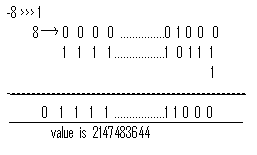## Sunday, February 8, 2009

### Java operators

Operators&Assignments:
• increment/decrement
• shift
• arithmetic
• equality(==,!=)
• relational
• bit-wise
• short-circuit
• instanceof
• cast
• conditional(?)
• new
• [ ]

Increment& decrement operators:
• Post Increment ------> y=x++;
• Pre Increment ------> y=++x;
• Post Decrement ------> y=x--;
• Pre Decrement ------> y=--x;

Example: int x=4;
int y=x++;
System.out.println(y); //4 .unexpecompile time errord type.
System.out.println(x); //5

Example: int y=++4;
System.out.println(y); //Compile time error

• Increment or decrement operators, we can apply Only for variables not for constant expressions. Violation leads to Compile time error saying unexpecompile time errord type Required: variable but found=value.
Ex: int x=4;
int y=++(++x);
System.out.println(y); //COMPILE TIME ERROR
• we can apply increment/decrement operators for variables only one time. Ie . nesting of increment/decrement operators are not allowed.
Ex: int x=y;
final int x=y;
x++;--- [x=x+1] x++;
System.out.println(x); //5
System.out.println(x); //COMPILE TIME ERROR
• Increment/decrement operators, we can’t apply for the final variables. Violation leads to compile time error saying can’t assign a values to final.
Ex: double d=10.5;
d++; System.out.println (d); //1.5
• Increment/decrement operators, we can apply for floating point data types.
Ex: byte b=10;
b=b+1;
System.out.println (b); //compile time error
Possible loss of precession
found: int , required :byte

Ex: byte b=10;
b=b++;
System.out.println (b); //11
i.e automatic typecasting takes place internaly

Ex: byte a=10;
byte b=20;
byte c=a+b;
System.out.println (c); //compile time error
• if we can perform any arithmetic operation between any 2 variables a and b the result is always max (int, type a, type b )
byte + short=int
char + char=int
int + char=int
int + long=long
doubles + byte=double

Arithmetic OPERATORS:-( + , - , * , / , % )
byte a=10
byte b=20
byte c=a+b; //compile time error plp req:byte
• If we can perform arithmetic operators b/w any two operands a and b , the result type is, max(int, type a, type b)
Ex: int a=10+’a’ => 10+97=107
double b=10+12.5 => 10.0+12.5=>22.5;
• For representing infinity, there are no constant ; define in the integer class. Hence 0/0 results is sum time exception saying arithmetic exception
System.out.println(10/0); //Runtime Exception by zero .
System.out.println(10.0/0); //infinity
System.out.println(10.0f/0); //infinity
• But in the float and double classes for representing infinity constants are defined.
System.out.println (Float. POSITIVE_INFINITY); // infinity
System.out.println( Float NEGATIVE_ INFINITY); //-infinity

final float postive_ infinity ,

final float negative _ infinity are the constants contain in float class.
0/0 is undefined in the Integer class for representing undefined results there is no constant available . Hence 0/0 results arithmetic exception.
0/0 →arithematic exception but in float and double classes for representing
undefined values there is a commands available . public static final float NaN.
NaN→not a number and public static final double NaN

Ex:
System.out.println(0.0 / 0) NaN
System.out.println(Math.sqrt(4)); 2.0
System.out.println(Math. Sqrt(-4)); NaN.
System.out.println(10/0)→ Arithmatic exception
System.out.println(10.0 / 0)→ infinity
System.out.println(-10.0 /0→ - infinitz
System.out.println(0/0) → Arithematic Exception.
System.out.println(0.0/0 → NaN
System.out.println(-10/0) arithmatic exception
System.out.println(-10.0/0)→ -infinity,
System.out.println(-0.0/0)→ NaN.
Float. POSITIVE_INFINITY = = Float. POSITIVE_INFINITY; // true
Float . POSITIVE_INFINITY = =Double. POSITIVE_INFINITY. // true
Float . NaN = =Float. NaN.
Float. NaN >double. NaN }false
Float. NaN !=Float. NaN →true.
• if one are comparing NaN with any other , including NaN itself, the result is always false, except for not equal(!=)operator.
Arithmetic exception:

1. runtime exception (unchecked)
2. only in the cone of integer arithmetic.
3. / and % only there two operators result in Arithmetic exception.
String concatenation operation:-
‘+’ is the only operator in java which in overloaded. We can one for arithmetic addition and for string concatenation.
String a=”java”.
int b=30;
int c=20;
int d=10;
System.out.println (a+b+c+d);→java302010;
System.out.println(b+a+c+d);→ 30java2010
System.out.println(b+c+d+a); →60java
System.out.println(b+c+a+d);→50java10.

* If at least one operand is of string type then + acts as string concatenation operator other wise + acts as arithematic operator.

SHIFT OPERATORS(1.4 ONLY):-
Left shift (<<)
Right shift ( >>)
Unsigned /zero filling right shift operator ( >>>)
In order to get high resolution of pictures
For compression of data also used these shift operators frequently use in j2me edition.
Left Shift Operator:
a << b shift the bits of a ,b times to the left hand side.
Ex: 8 << 33 then 8 << 33 % 32 = 8 << 1 // result=16
Ex: 8 << - 31 then 8 << -31+32 = 8 << 1 // result= 16Right Shift Operator:
a>>b shift the bits of a ,b times to the right hand side by filling the left hand side bits with sign bit.
8 >> 1 --> 0000 …….100 = 4.
Filled with sign bit zero.
a >> b =a/2 power b for 8 >> 1 =8/2 power 1 =8/2=4.
If b < 31 then b=b+32
If b >31 then b= b% 32.
- 8 >> 1 ---> -8/2 power 1 = -8/2= -4.

Unsigned Right Shift Operator:
a>>> b , shift the bits of a, b times to the right hand side. By filling left most bits with zero always.If a is +ve ,then there is no difference between right shift and unsigned right shift operator.Except shift distance zero ,the result of unsigned right shift operator is always positive.
I few are applying shift operator between a and b the result type is always max(int type of a)Case1: byte b=8 >>1; // valid
Case2: int a=8;
byte b=a >> 1; // invalid
Case3: final int a=8;
byte b=a>>1; // valid
Case 4:long c=1;
byte b=8>>c; //invalid (as per max(int,type of a)
In a << ,>> ,>>> b ,If a and b ,both are compile time constants then the compiler won’t go for formula for the result type.If at least one in the variable ,either a or b then the compiler checks the type of the result which is max(int,type of a) , the type b never participated in the result .